Question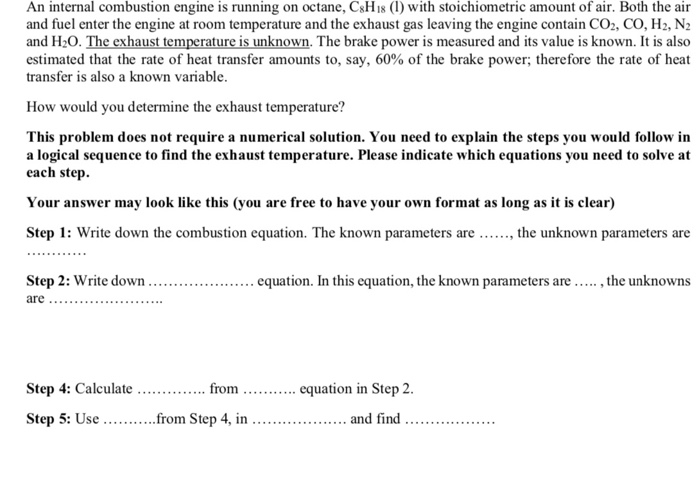Please thumbs up to appreciate our efforts If you have any doubt regarding this answer, please tell me. I'll be delightful to answer.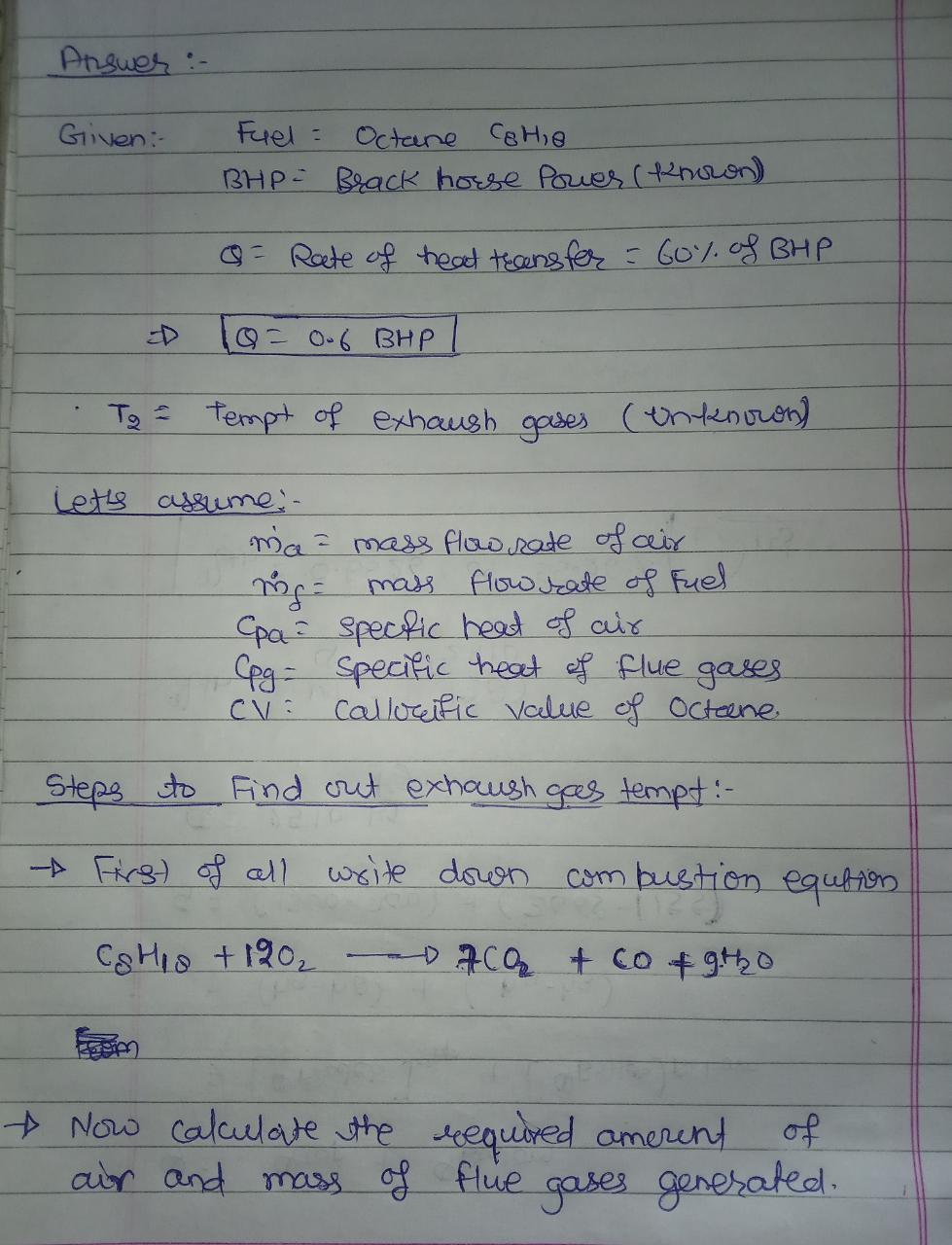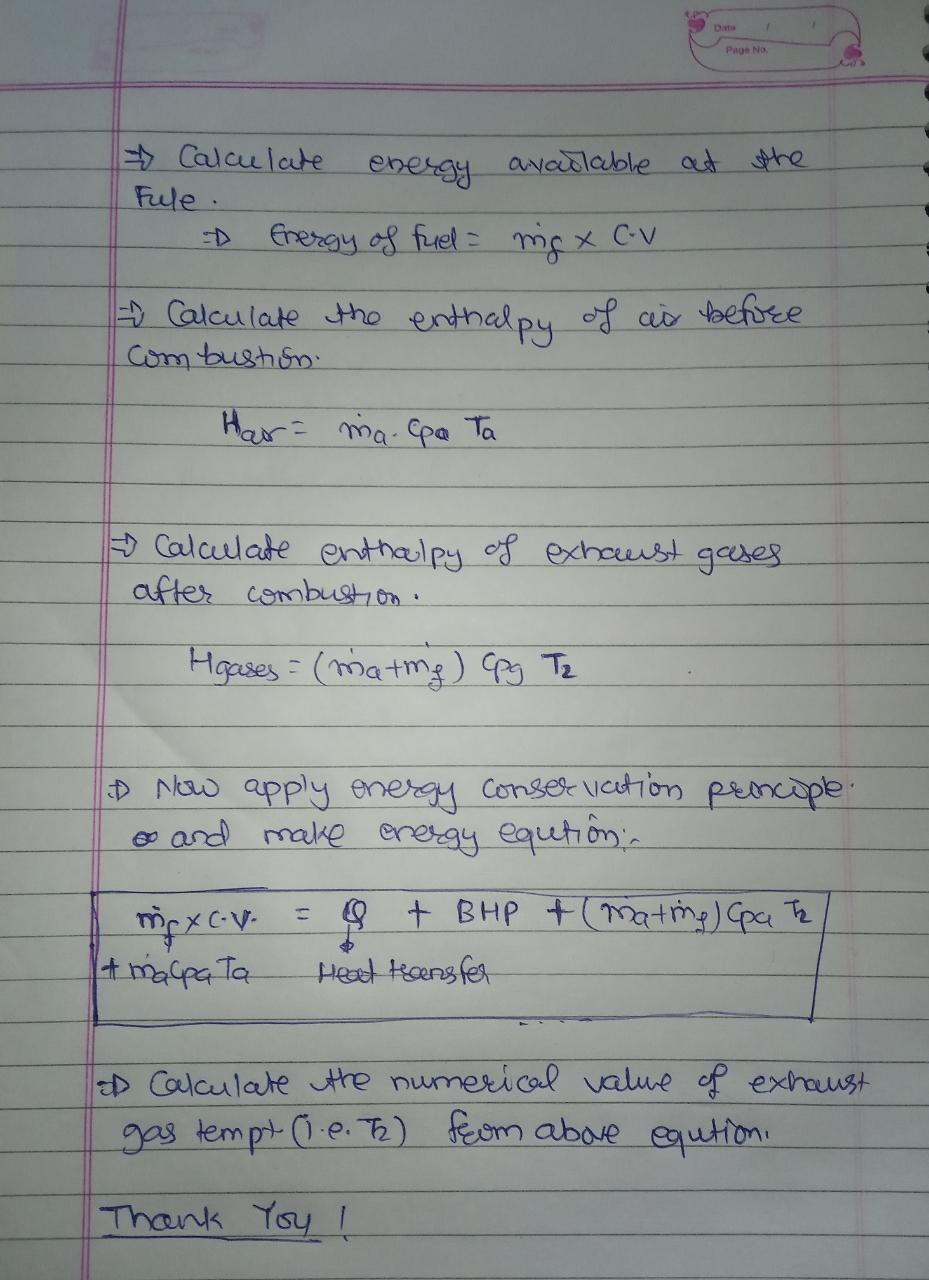#### Earn Coins

Coins can be redeemed for fabulous gifts.

Similar Homework Help Questions
• ### The heat of combustion of octane is -5430 kJ/mol. 1.000 g sample of octane (C8H18) is...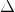The heat of combustion of octane is -5430 kJ/mol. 1.000 g sample of octane (C8H18) is burned in a bomb calorimeter at an initial temperature of 25.00ºC. The heat capacity of the calorimeter (also known as the “calorimeter constant”) is 837 J/ºC. Calculate E for the combustion of octane. (You will need a balanced chemical equation to find H) What is the final temperature of the calorimeter?

• ### Consider a 4-cylinder, 4-stroke square engine running on octane, C8H18 (l) at an equivalence ratio of...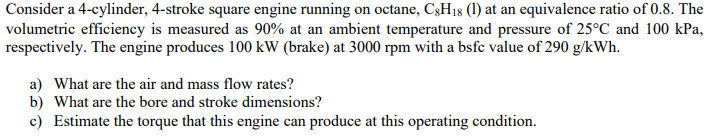Consider a 4-cylinder, 4-stroke square engine running on octane, C8H18 (l) at an equivalence ratio of 0.8. The volumetric efficiency is measured as 90% at an ambient temperature and pressure of 25C and 100 kPa, respectively. The engine produces 100 kW (brake) at 3000 rpm with a bsfc value of 290 g/kWh. Consider a 4-cylinder, 4-stroke square engine running on octane, CH18 (1) at an equivalence ratio of 0.8. The volumetric efficiency is measured as 90% at an ambient temperature...

• ### QUESTION 1 (25%) Consider a 4-cylinder, 4-stroke square engine running on octane, C8H18 (1) at an...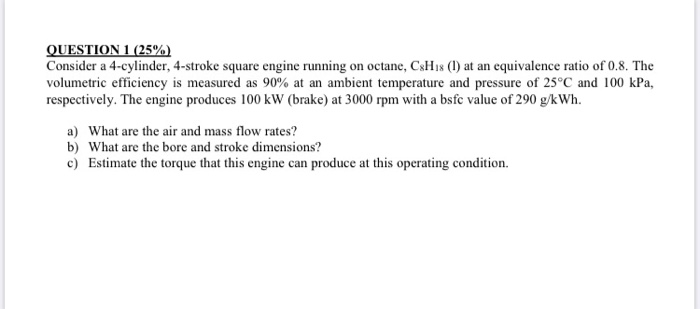QUESTION 1 (25%) Consider a 4-cylinder, 4-stroke square engine running on octane, C8H18 (1) at an equivalence ratio of 0.8. The volumetric efficiency is measured as 90% at an ambient temperature and pressure of 25°C and 100 kPa, respectively. The engine produces 100 kW (brake) at 3000 rpm with a bsfc value of 290 g/kWh. a) What are the air and mass flow rates? b) What are the bore and stroke dimensions? c) Estimate the torque that this engine can...

• ### QUESTION 1 (25%) Consider a 4-cylinder, 4-stroke square engine running on octane, C8H18 (1) at an...QUESTION 1 (25%) Consider a 4-cylinder, 4-stroke square engine running on octane, C8H18 (1) at an equivalence ratio of 0.8. The volumetric efficiency is measured as 90% at an ambient temperature and pressure of 25°C and 100 kPa, respectively. The engine produces 100 kW (brake) at 3000 rpm with a bsfc value of 290 g/kWh. a) What are the air and mass flow rates? b) What are the bore and stroke dimensions? c) Estimate the torque that this engine can...

• ### Consider an internal combustion engine like that used in automobiles. The most common fuel used here...

Consider an internal combustion engine like that used in automobiles. The most common fuel used here is gasoline which is a mixture of hydrocarbons, and an octane rating is given for standardization. Therefore, we will approximate gasoline as being composed of the hydrocarbon, octane, C8H18. Another fuel that is common in racing applications is nitromethane, CH3NO2, used in top fuel dragsters. Let’s consider the combustion of each fuel with oxygen in an internal combustion engine. Let the 8-cylinder engine displacement...

• ### (a) Consider methane is burned in an internal combustion engine using 200% theoretical air. Locate the...

(a) Consider methane is burned in an internal combustion engine using 200% theoretical air. Locate the following unknown parameters: I. Air/fuel ratio on mass basis II. Composition of products III. Dew point temperature of the products

• ### 3. A rich fuel-air ratio of propane in burned in an internal combustion engine. The analysis...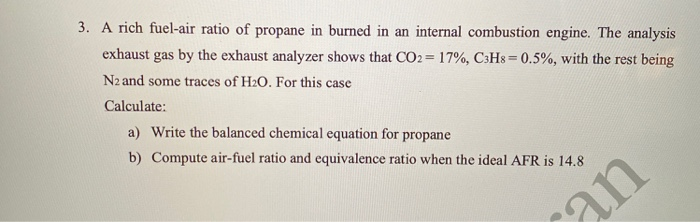3. A rich fuel-air ratio of propane in burned in an internal combustion engine. The analysis exhaust gas by the exhaust analyzer shows that CO2 = 17%, C3H8=0.5%, with the rest being N2 and some traces of H20. For this case Calculate: a) Write the balanced chemical equation for propane b) Compute air-fuel ratio and equivalence ratio when the ideal AFR is 14.8 ran

• ### 2. Analyze an Audi 3.0-liter TDI V6 Biturbo diesel engine using an air-standard Diesel cycle that...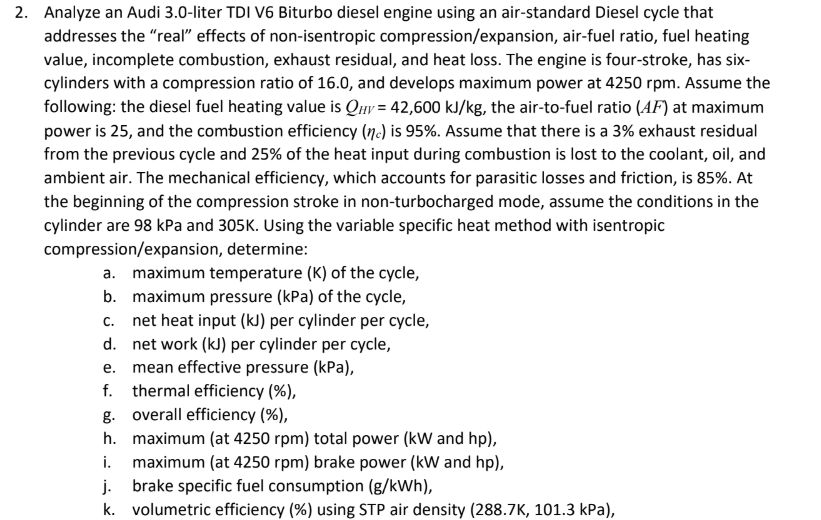2. Analyze an Audi 3.0-liter TDI V6 Biturbo diesel engine using an air-standard Diesel cycle that addresses the "real" effects of non-isentropic compression/expansion, air-fuel ratio, fuel heating value, incomplete combustion, exhaust residual, and heat loss. The engine is four-stroke, has six cylinders with a compression ratio of 16.0, and develops maximum power at 4250 rpm. Assume the following: the diesel fuel heating value is Quv 42,600 kJ/kg, the air-to-fuel ratio (AF) at maximum power is 25, and the combustion efficiency...

• ### A2. Ethylene vapour C2H4 at 27°C is burned in a combustion chamber with 15% excess air, which is also supplied at 27 °C. The temperature and pressure of the products of combustion are 640 °C and 1.1...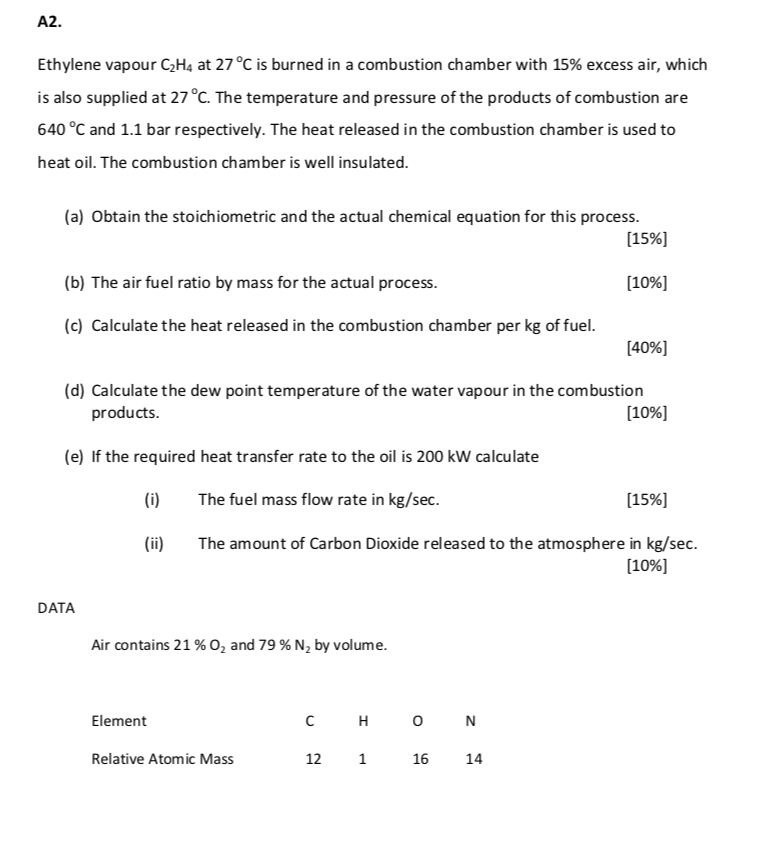A2. Ethylene vapour C2H4 at 27°C is burned in a combustion chamber with 15% excess air, which is also supplied at 27 °C. The temperature and pressure of the products of combustion are 640 °C and 1.1 bar respectively. The heat released in the combustion chamber is used to heat oil. The combustion chamber is well insulated (a) Obtain the stoichiometric and the actual chemical equation for this process   (b) The air fuel ratio by mass for the...

• ### An air-breathing gas-turbine jet engine operates at SSSF with complete combustion of 1 kmol / s of liquid ethanol fuel (C₂H₅OH)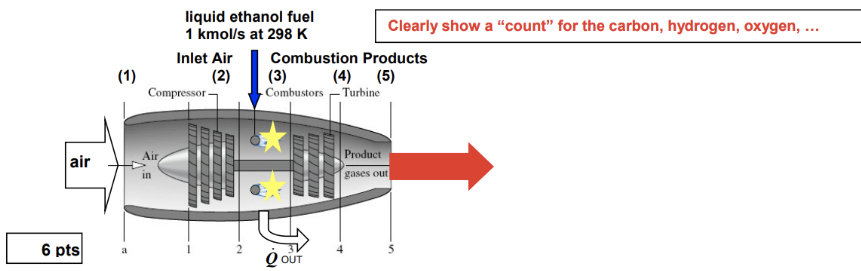An air-breathing gas-turbine jet engine operates at SSSF with complete combustion of 1 kmol / s of liquid ethanol fuel (C₂H₅OH). The given air-fuel ratio is 33 kg-air/kg-fuel. The constant pressure combustion process (2 → 3) results in a small amount of heat transfer out to the surroundings. Assume the inlet air is composed of 21 % O₂ and 79 % N₂(1 O₂+3.76 N₂)FIND the theoretical air-fuel ratio, AFTHEORETICAL= _______ kg-air/kg-fuelFIND the concentration of CO₂ in the exhaust gas on...